# Noise requirements for ADC power supplies

Other Parts Discussed in Post: TPS717

So far in this blogs series, we’ve looked at the power supply rejection ratio (PSRR) of the ADC and the PSRR requirements for the prior power stage to ensure minimal noise.  Before further analyzing the power supply, we need to understand the impact of supply noise on the ADC.

In this blog, we will look into the thermal and flicker noise requirements for the ADC power supply and derive its minimum requirements to ensure excellent Signal-to-Noise ratio (SNR).

Given the SNR from an ADC, we can calculate the root mean square (RMS) input-referred noise with Equation 1: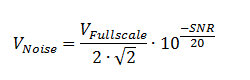where:

VNoise is the input referred voltage noise

SNR is the Signal-to-Noise Ratio in dB

VFullscale is the Full scale of the considered ADC

The origin of the noise is not as important as the bandwidth which it is referred to, so considering that the ADC analog bandwidth (ABW) can be modeled as a first order Butterworth filter, the noise-power bandwidth (NPBW) can be expressed as Equation 2: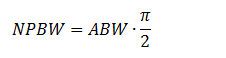Note that this approach, while accurate from the perspective of the complete analog bandwidth, is incorrect from the usage of an ADC.  When using an ADC, you will always be operating in one of the Nyquist bands most often the first Nyquist band from DC to half the switching frequency ( ), so the noise will always be folded in a frequency band of  bandwidth.

We can then calculate noise density using Equation 3: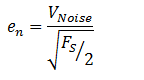Where FS is the ADC clock

As an example, let’s start with an ideal ADC with noise being only due to quantization.  The SNR can be expressed as Equation 4.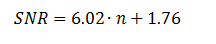where n is the number of bits.

Limiting ourselves to the quantization noise allows us to develop a worse-case requirement for an ideal ADC power supply and give us a reference.

Table 1 reflects the calculation of the SNR and input-referred input voltage noise density for various ideal ADCs using equations 1, 3 and 4.

 VFullscale = 2Vpp Input-referred noise density for a given FS/2 bandwidth # of bits SNR 1kHz (nV/√Hz) 10kHz (nV/√Hz) 100kHz (nV/√Hz) 500kHz (nV/√Hz) 1MHz (nV/√Hz) 5MHz (nV/√Hz) 10MHz (nV/√Hz) 50MHz (nV/√Hz) 100MHz (nV/√Hz) 8 50 4472.1 3162.3 1414.2 1000.0 447.2 316.2 10 62.06 7888.6 2494.6 1115.6 788.9 352.8 249.5 111.6 78.9 12 74.12 6223.0 1967.9 622.3 278.3 196.8 88.0 62.2 27.8 19.7 14 86.18 1552.4 490.9 155.2 69.4 49.1 22.0 15.5 6.9 4.9 16 98.24 387.3 122.5 38.7 17.3 12.2 5.5 3.9 1.7 1.2 18 110.3 96.6 30.5 9.7 4.3 3.1 20 122.36 24.1 7.6 2.41 22 134.42 6.0 1.9

Table 1: Equivalent input-referred voltage-noise density for ideal ADCs in the first Nyquist band

In table 1, we have on the left, the number of bits for the ideal ADC considered in the first column.  In the 2nd column, we have the SNR for an ideal ADC.  This is coming directly from equation 4.  Each of the subsequent columns correspond the ADC equivalent input noise referenced to the Nyquist frequency in the 2nd row.

Having a voltage noise density, we can then calculate the noise contributed by the power supply to the signal chain signal.

Once again using the ADC3444 as an example, we established earlier that the analog supply was the most sensitive and that the minimum worse-case PSRR was 16dB.  See Figure 1 for typical values and the “ADC Power Supply Rejection Ratio” blog post for the discussion.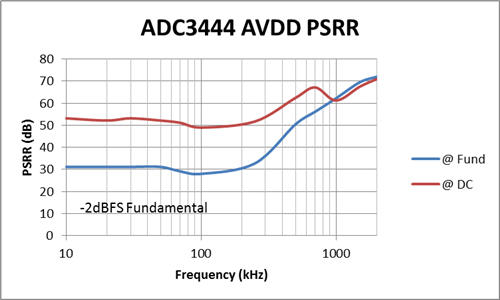Figure 1: ADC3444 analog VDD (AVDD) PSRR vs. frequency

The next step is to determine the allowable noise from the power supply starting first with an ADC having 0dB of PSRR to calculate the worst case.  For this section, let’s consider several LDOs, with noise of 100µVRMS, 50 µVRMS, 20 µVRMS, 10 µVRMS and 5 µVRMS.  These numbers correspond approximately to TI DC-DC converters or LDOs without noise specification (100 µVRMS); the TPS737 LDO (50 µVRMS); the TPS7A8101; the TPS74x LDO family (20 µVRMS); the TPS717 LDO (10 µVRMS) and the TPS7A83, LP5907, TPS7A47 LDOs (5 µVRMS).

The noise (or in this case the voltage-noise density) adds in RMS fashion as shown in Equation 5.With a PSRR of 0dB, Table 1, with the noise integration bandwidth for the power supply set as 10Hz to 100kHz, can be rewritten as Table 2:.

 VFullscale = 2Vpp Noise density Total noise for select Power Supply Noise # of bits 50MHz (nV/√Hz) 5µVRMS (nV/√Hz) 10µVRMS (nV/√Hz) 20µVRMS (nV/√Hz) 50µVRMS (nV/√Hz) 100µVRMS (nV/√Hz) 8 316.2 316.6 317.8 322.5 353.6 447.2 10 78.9 80.5 85.0 101.1 176.7 325.9 12 19.7 25.2 37.2 66.2 159.3 316.9 14 4.9 16.6 32.0 63.4 158.2 316.3 16 1.2 15.9 31.6 63.3 158.1 316.2

Table 2: Combined ADC and power supply noise for a 50MHz Nyquist bandwidth

In table 2, we have selected the 50MHz Nyquist band from Table 1, shown in column 2 and have kept the first column as the ADC number of bits.  Each additional column now contains the resulting of the ADC and the noise power supply referred in row 2.

In Table 3, we move one step further and express the results from table 2 back into an SNR number that includes both the ADC noise and the power supply noise.

Now we can easily the combined SNR to the nominal SNR.  For an ideal 8bit ADC, a 50µVRMS LDO would prove sufficient.  The TPS7A81 or TPS717 LDO would be a perfect match here.  Keep in mind that the noise number provided above are but a coefficient to the output voltage and can be expressed as µVRMS/VOUT.  So for a 3.3V output voltage a lower noise LDO would be required.

High bit-count ADCs have even more stringent demand on the power supply.

 Fullscale = 2Vpp Noise density Total noise for select Power Supply Noise # of bits SNR for 50MHz baseband (dB) 5µVRMS (dB) 10µVRMS (dB) 20µVRMS (dB) 50µVRMS (dB) 100µVRMS (dB) 8 50.0 49.99 49.96 49.83 49.03 46.99 10 62.1 61.9 61.4 59.9 55.1 49.7 12 74.1 72.0 68.6 63.6 56.0 50.0 14 86.2 75.6 69.9 64.0 56.0 50.0 16 98.2 76.0 70.0 64.0 56.0 50.0

Table 3: Equivalent ADC SNR once including power supply noise for 50MHz baseband

Fortunately for us, the ADCs have some PSR as well that would need to be considered in the component selection.

The ADC3444 AVDD has two PSR curve, one around DC with high PSR and one around the signal tone, which is much lower.  In the case of the AVDD for the ADC3444, there are two mode of entry for the power supply noise to degrade the ADC SNR.  The dominant term of the two modes is the noise coming around the signal tone as it will be twice the power supply noise attenuated by the 28dB nominal PSR.

The term around DC will not have any multiplying coefficient and will have a PSR of 50dB.

This leads us to table 4a and 4b:

 ADC input-referred noise density 20.2 nV/rtHz (73.9dB SNR) ADC worst case PSRR (dB) 50 dB Power supply noise Combined Noise (nV/√Hz) Combined SNR (dBFS) 5µVRMS 20.2 73.9 10µVRMS 20.1 73.9 20µVRMS 20.2 73.9 50µVRMS 20.2 73.9 100µVRMS 20.2 73.9

Table 4a: Noise added around DC by the power supply

 ADC input-referred noise density 20.2 nV/rtHz (73.9dB SNR) ADC worst case PSRR (dB) 28 dB Power supply noise Combined Noise (nV/√Hz) Combined SNR (dBFS) 5µVRMS 20.2 73.9 10µVRMS 20.2 73.9 20µVRMS 20.4 73.8 50µVRMS 21.2 73.5 100µVRMS 23.8 72.5

Table 4b: Noise added around the signal tone by the power supply

To conclude, the power supply flicker noise can degrade significantly the performance of an ADC and careful selection of the LDO is required.  In the case of the ADC3444, the total power supply noise should not exceed 20µVRMS for minimal impact on the ADC SNR specifications.

This was demonstrated here with a high speed pipeline converter, but it also applies to all ADCs, Delta-Sigma and SAR architectures.

In this blog, I have shown the impact of power supply noise on sensitive power-supply rails of the ADC.  In my upcoming blogs, I will explore post-filtering strategies and finally develop a full, high performance power supply solution for the ADC3444.Parents
• Hi David,

The maximum power supply noise depends on how much SNR degradation can be accepted in your application.

A target of 100uVrms is valid for the entire power supply noise.  If the ADC supply pin has higher PSRR or less variation of PSRR over temperature and process, the power supply noise requirements will be reduced.

The LDO selection may be adjusted as well for the need.  Keep in mind that most LDO noise only consider the flicker noise (to 100kHz) and ignore the gain applied to the noise when the output voltage is raised as well as the wideband noise contribution.

Best regards,

Xavier

Comment
• Hi David,

The maximum power supply noise depends on how much SNR degradation can be accepted in your application.

A target of 100uVrms is valid for the entire power supply noise.  If the ADC supply pin has higher PSRR or less variation of PSRR over temperature and process, the power supply noise requirements will be reduced.

The LDO selection may be adjusted as well for the need.  Keep in mind that most LDO noise only consider the flicker noise (to 100kHz) and ignore the gain applied to the noise when the output voltage is raised as well as the wideband noise contribution.

Best regards,

Xavier

Children
No Data